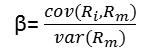# Capital Asset Pricing Model in finance with python

### What is CAPM?

CAPM  means capital asset pricing model and it is financial model.It describes the relationship between  expected return and risk of security.
We will start very popular,one factor model (The Capital Asset Pricing Model or CAPM) .The CAPM assumes the existence of one riskfree asset in which every agent can invest any amount which earns the risk free rate .When stock returns are normally distributed,price of single stock can be elegantly expressed in relationship to a broad market index, the relationship is genarally expressed by a measure for a comovment of a single stock with the market index called beta.
CAPM  is represented by the following equation:

Here,

Beta can be interpreted as the level of the asset return's sensitivity as compare to the market in general.
Some possible examples:
If Beta <=-1 ,the asset moves in the opposite direction as the benchmark and in a greater amount than the negative of the benchmark.
If -1<Beta <0 ,the asset moves in the opposite direction to the benchmark.
If Beta =0,there is no correlation between the asset's price movement and the market benchmark.
If 0<Beta<1,the asset moves the same direction as the market but amount is smaller.
If Beta=1, the asset and the market are moving in the same direction by the same amount.
If Beta >1, the asset moves in same direction as the market,but amount is grater.## CAPM is also called One Factor Model.

How to apply CAPM  and help investment with python?
Import libraries :
import panda as pd
import yfinance as yf
import statsmodels.api as sm
First specified the assets(Reliance and Nifty50)
We wanted to use  and time frame
Python code:
RISKY_ASSET = 'RELIANCE.NS'
MARKET_BENCHMARK = '^NSEI'
START_DATE = '2018-08-01'
END_DATE = '2021-09-16'
Data from yahoo finance
start=START_DATE,
end=END_DATE,
progress=False)

Convert data daily into monthly
MARKET_BENCHMARK: 'market'}) \
.resample('M') \
.last() \
.pct_change() \
.dropna()
Calculate Beta using covariance

### CORRELATION DEFINITIONS

covariance = X.cov().iloc[0,1]
benchmark_variance = X.market.var()
beta = covariance / benchmark_variance

## The result of code beta =1.0546919715802414

These results indicate that the beta (denoted as nifty50)
is equal to 1.05..,which means Reliance's returns are 5 % more
volatile than the market .The value of the intercept is relatively
small and statistically insignificant at the 5% significance level.
Estimate CAPM as linear regression code in python
y = X.pop('asset')
capm_model = sm.OLS(y, X).fit()
capm_model = sm.OLS(y, X).fit() print(capm_model.summary())
The results of estimating CAPM model.

### Regration Analysis Used for

OLS Regression Results
============================================================================== Dep. Variable: asset R-squared: 0.470 Model: OLS Adj. R-squared: 0.455 Method: Least Squares F-statistic: 31.00 Date: Fri, 17 Sep 2021 Prob (F-statistic): 2.87e-06 Time: 13:18:11 Log-Likelihood: 45.743 No. Observations: 37 AIC: -87.49 Df Residuals: 35 BIC: -84.26 Df Model: 1 Covariance Type: nonrobust ============================================================================== coef std err t P>|t| [0.025 0.975] ------------------------------------------------------------------------------ const 0.0089 0.012 0.737 0.466 -0.016 0.034 market 1.0547 0.189 5.568 0.000 0.670 1.439 ============================================================================== Omnibus: 0.982 Durbin-Watson: 1.583 Prob(Omnibus): 0.612 Jarque-Bera (JB): 0.394 Skew: -0.234 Prob(JB): 0.821 Kurtosis: 3.193 Cond. No. 15.9 ==============================================================================

We separted the target(Reliance's stock returns) and the
features(Nifty50 returns) using pop method of a panda's
data frame .we added the constant to the features with
to the regression is to investigate wether after estimating the
model-the intercept is zero.If it is positive and significant
it means that assuming the CAPM model is true, the asset or portfolio
generates abnormally high risk adjested return.There are two
possible implications either the market is inefficient
or there are some other undiscovered risk factor that should
be included in the model. This issue is known as hypothesis problem.
we get OLS regression,we could see that the coefficient
by the market variable is the CAPM beta is equal to the beta
that was calculated using the covariance between the asset and
the market.

#### Other Examples

There are so many ways to get forecast stock price. I am going to have separated the target (TCS.NS's stock returns) and the features (NSEIreturns) using the pop method of a pandas DataFrame. Afterward, we added the constant to the features (effectively adding a column of ones) with the add_constant function.
The idea behind adding the intercept to this regression is to investigate whether—after
estimating the model—the intercept (in the case of the CAPM, also known as Jensen's
alpha) is zero. If it is positive and significant, it means that– assuming the CAPM model is true—the asset or portfolio generates abnormally high risk-adjusted returns. There are two possible implications—either the market is inefficient or there are some other undiscovered risk factors that should be included in the model. This issue is known as the joint hypothesis problem.
Beta of TCS is 0.591020194859077

### How do you write  CAPM code  in python?

There are number of way to write capm code in python.I have discribed all process with link.